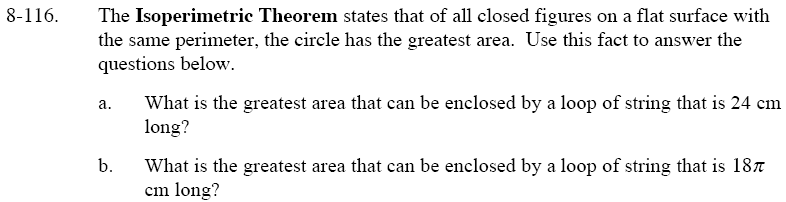Home > GC > Chapter 8 > Lesson 8.3.3 > Problem8-116

8-116.
1. The Isoperimetric Theorem states that of all closed figures on a flat surface with the same perimeter, the circle has the greatest area. Use this fact to answer the questions below. Homework Help ✎

1. What is the greatest area that can be enclosed by a loop of string that is 24 cm long?

2. What is the greatest area that can be enclosed by a loop of string that is 18π cm long?The 24 cm loop of string is the circumference of the circle. To calculate r use the circumference formula, 2πr = 24.

Solve for r, then substitute it into the equation: Area of a circle = πr²

Area ≈45.84 cm²

The only difference between the two parts of this problem is that the length of the loop of string has been changed.
Therefore, follow the same steps used in part (a) to find the answer to part (b).